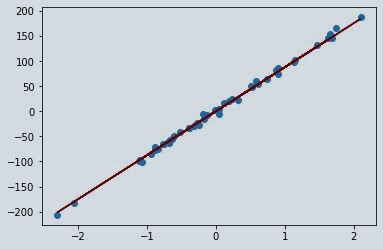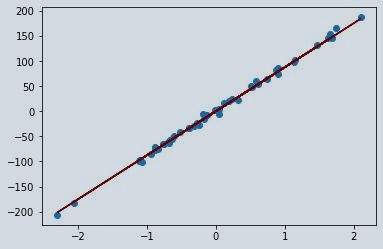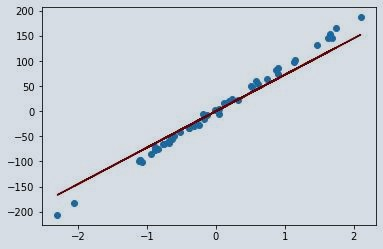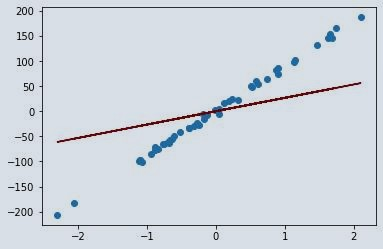Table of Content

## Introduction

Ridge regression is a model which is used to analyze data which suffers multicollinearity. When this multicollinearity occurs, least squares are unbiased and the variances are large making the predicted values to be far from the actual values. The ridge regression standard error can be reduced by adding a degree to the bias. The effect of multicollinearity can result to wrong estimate of regression coefficient and also can increase the standard errors of regression coefficient. It performs L2 regularization.

## Origin of Multicollinearity

1. Data Collection- It can cause multicollinearity
2. Outliers- Extreme values can cause multicollinearity
3. Over defined model – Variable count are more than the observation count

## Regression Model

The basic equation for any type of regression is

Y=XB + e

In which,

Y represent dependent variable,

X represents independent variable,

B represent regression coefficient which will be calculated later and

lastly e represents errors which are residuals.

## Standardization

While performing ridge regression the first step is to standardize the variables in dataset on which you are working. Standardization is done by subtracting their means and dividing it with standard deviation. This is performed on both dependent and independent variables which are there in the dataset. All the calculations done are based on standardise value. When we set the final regression coefficient, the standardized variables are converted back to the original scale.

## Ridge Regression Assumptions

The assumption in ridge regression is same as assumption in linear regression which is constant variance, independence and linearity. Normality should not be assumed as ridge regression does not provide confidence limit.

## Implementing Ridge Regression using Python

For better understanding of ridge regression, we will look at the following example performed in python.

1. First Import all the required libraries
```    from sklearn.datasets import make_regression
from matplotlib import pyplot as pltplt
import numpy as npnp
from sklearn.linear_model import Ridge

```
1. For explaining purpose, we will create a sample dataset using scikit -learn which will match the regression.
```    L, g, coefficient = make_regression(
n_samples=50,
n_features=1,
n_informative=1,
n_targets=1,
noise=5,
coef=True,
random_state=1
)

```
1. Regularization

Here we will define the hyperparameter which is alpha. alpha defines the strength of the regularization. the larger the value of alpha the greater the strength of the regularization. it means that the bias of the model will be high when the alpha value will be high. when we set the alpha value to 1 i.e., alpha =1 then the model will act similar to linear regression model.

```    alpha_alpha = 1
n, m = L.shape
Id = npnp.identity(m)
z = npnp.dot(npnp.dot(npnp.linalg.inv(npnp.dot(L.T, L) + alpha_alpha * Id), L.T), g)

```

and also we solve for z using the equation mentioned above.

when we compare z and actual coefficient which is used in generating data, we can see that they are not equal to each other but are very close to each other

```    z
array([87.37153533])

coefficient
array(90.34019153)
```

1. Now let see how the regression line will fit the data.
```    pltplt.scatter(L, g)
pltplt.plot(L, z*L, c='maroon')```

1. Now we will create and train instance of ridge class.
```    rrrr = Ridge(alpha=1)
rrrr.fit(L, g)
z = rrrr.coef_
z
array([87.39928165])

```

we get the same value of z where we solved it for linear algebra. So, the regression line will be similar to the one above.

```    pltplt.scatter(L, g)
pltplt.plot(L, z*L, c='maroon')```

To see the effect of regularization hyperparameter i.e., alpha we can set the alpha value to whichever value we want to avoid overfitting or under fitting. Here I am taking alpha value as 11 and we will visualise it and will compare it to the previous one as well.

```    rrrr = Ridge(alpha=11)
rrrr.fit(L, g)
z = rrrr.coef_
pltplt.scatter(L, g)
pltplt.plot(L, z*L, c='maroon')```

As we can see the regression line is no longer a perfect line for the dataset. we can say that ridge regression model has a higher bias compared to the one where we have taken alpha value as 1. For better understanding let’s take alpha value as 111 and lets visualise it. So, we can observe and compare the results.

```    rrrr = Ridge(alpha=111)
rrrr.fit(L, g)
z = rrrr.coef_
pltplt.scatter(L, g)
pltplt.plot(L, z*L, c='maroon')```

## Conclusion and Summary

When the alpha value moves towards positive infinity, the regression line will move towards a mean of 0. This happens because it minimizes the variance across different dataset. In this article, we studied ridge regression and had a look on the origin of multicollinearity. We also studied regression model and assumption of ridge regression as well. At last, we implemented ridge regression on the dataset and found out that it has better performance as compare to the simple regression model. You can learn more about linear regression by reading this article on How to implement Linear Regression in Python from Scratch.